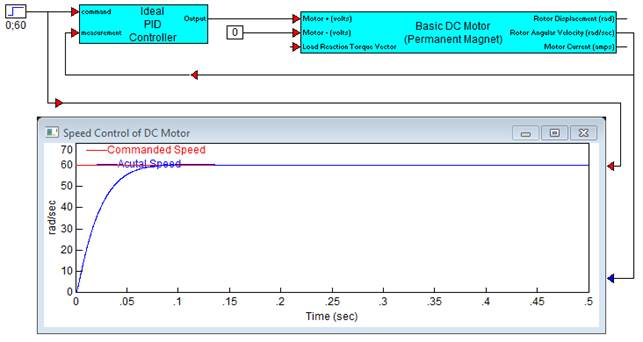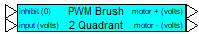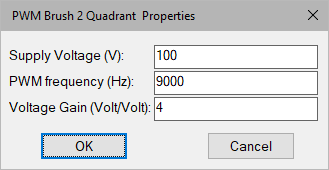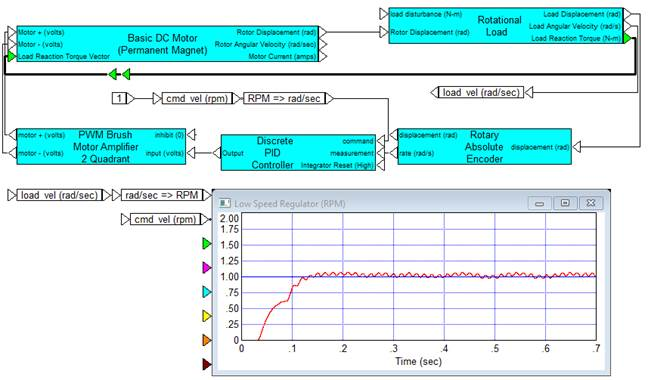#### Park TransformCategory: eMotors > Other Tools > Transforms

The Park Transform block transforms orthogonal signals and systems components [alpha beta] to direct and quadrature components [d q] given a particular rotation angle, θ.

Transformation is given by:#### Example

Diagram name: Park Transform

Location: Examples > eMotors > Transforms

This example shows the transformation of a 3-phase source modulated by a converging exponential envelope, first transformed to orthogonal components alpha, beta by Clarke’s transform, then to the rotating DQ frame by Park’s transformation. Here the input angle to Park’s transformation is the angle of rotation according to the 3-phase signal’s frequency. The result is the direct component tracing the signal envelope, and the quadrature signal at 0.